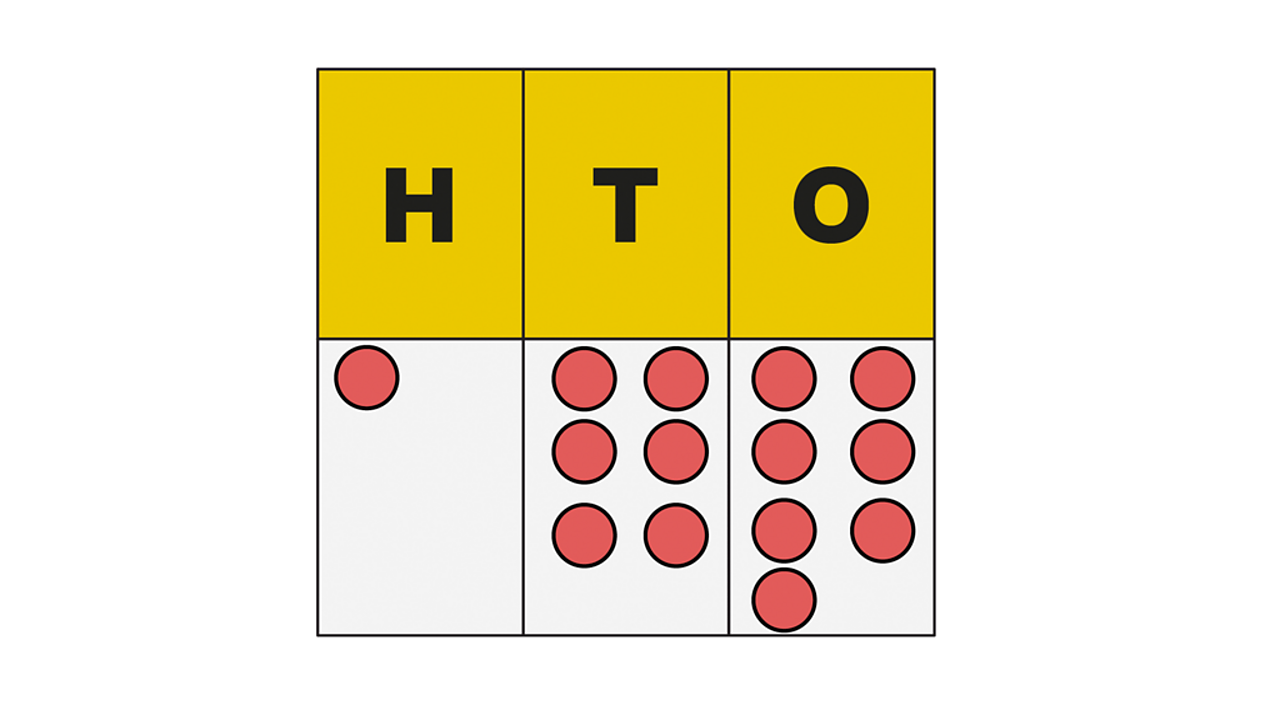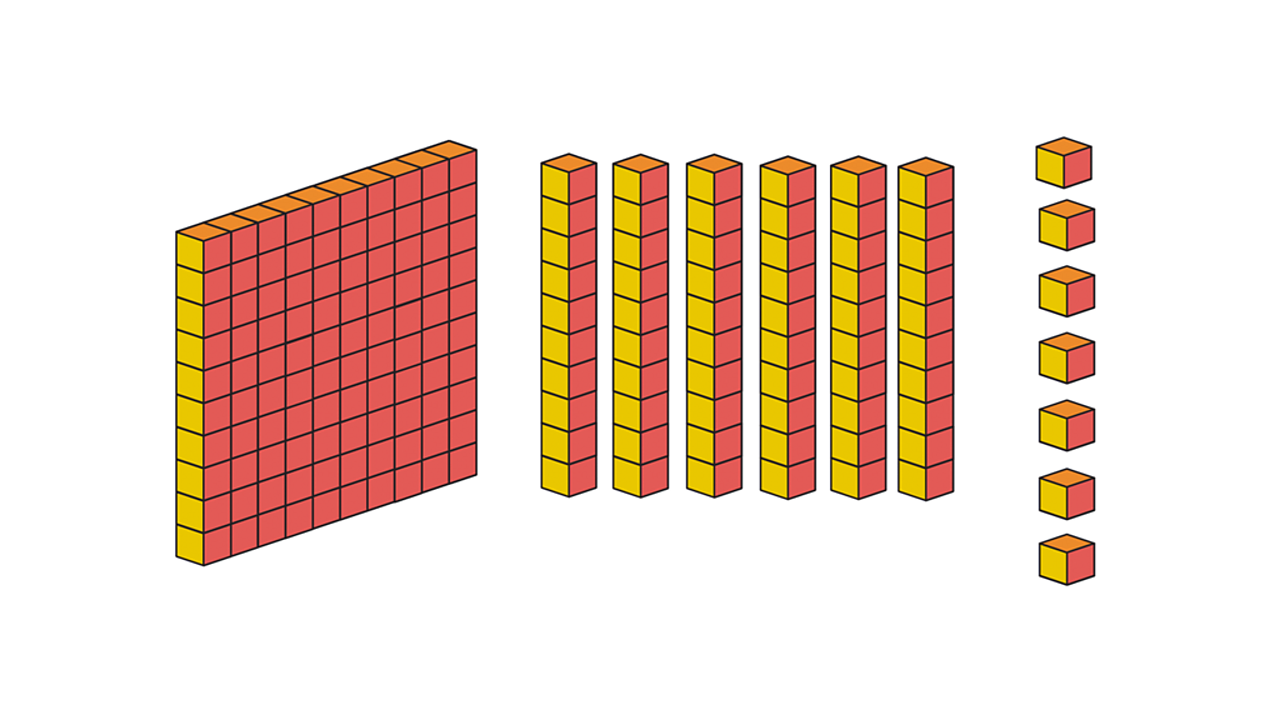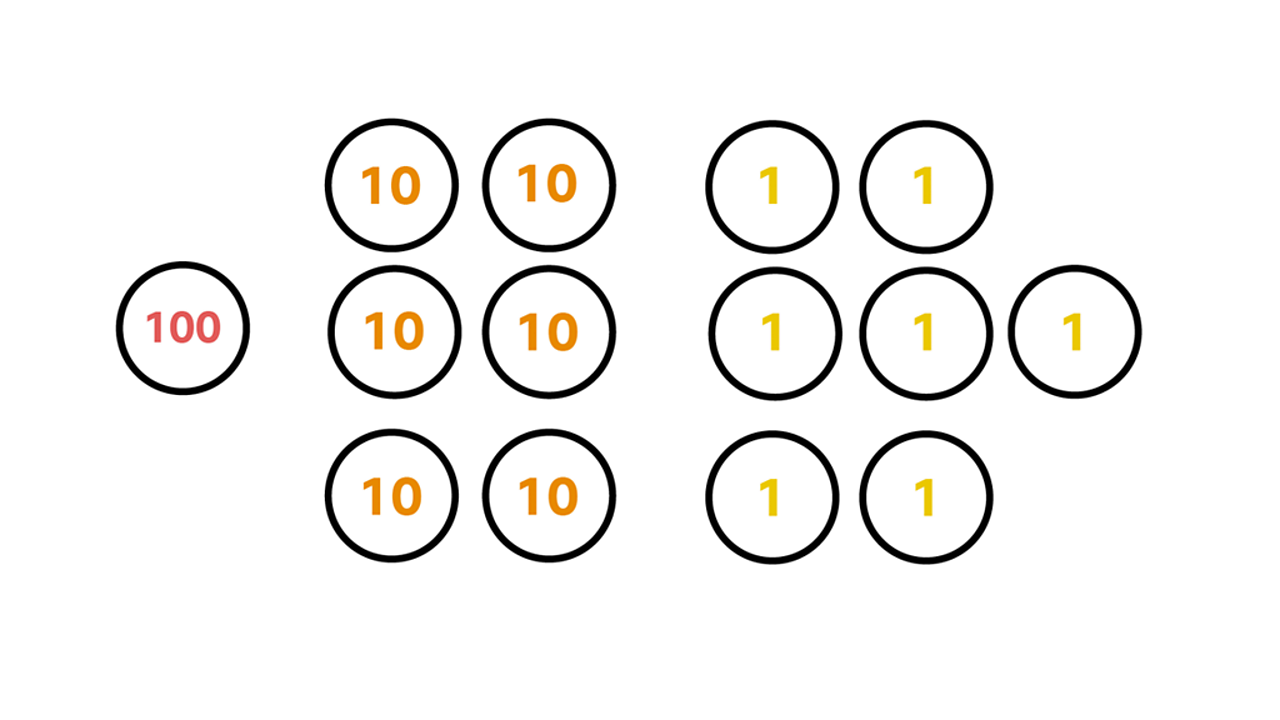# Numbers to 1,000

## Learning focus

Learn how to read and recognise numbers to 1,000.

This lesson includes:

• one quiz
• one video
• two interactive activities
• one worksheet

# Quiz

Check how well you know this topic. Take the quiz to practise reading and recognising numbers up to 1,000.

# Learn

Place value is really important when building up numbers. The order the digits are written in a number determine its value.

To remind you what place value is, here's an introduction with some dinosaur friends.

A number can be made of more than one digit. So we use place value headings, like ones, tens, hundreds and thousands, to help us understand the value of each digit.

## Example 1

How do you represent the number 167 with a place value chart?

The number 167 is made of the digits 1, 6 and 7.

We can use a place value to chart to express the number 167 like this:

HundredsTensOnes
167

In other words, 1 hundred, 6 tens and 7 ones.

This number can also be represented in other ways without using numbers. Each pictorial representation in the slideshow below shows 167.Place value chartA place value chart can also use dots to represent the digits. The dots can be counted to find 1 hundred, 6 tens and 7 ones.1 of 3Base 10 blocksBase 10 blocks can also be used to represent a number. A 10 x 10 square block represents a hundred. A 10 x 1 row represents a ten. An individual block represents a one.2 of 3Place value countersThe number can also be represented with counters. You can have a counter for each hundred, each ten and each one.3 of 3

Remember, if the digits were put in a different order, the number would change completely.

## Example 2

Let's rearrange the digits 1, 6 and 7 into 671 to see how it changes the number.

### Place value counters

The same digits have been used but they are in a different order. Their value completely changes.

## Example 3

Ellie wants to show the number 345 using place value counters. Can you help her?

The first thing you need to do is partition the number. That's 3 hundreds, 4 tens and 5 ones.

Now put them together to represent 345:

# Practise

## Activity 1

Dinosaur digits

Click on the image below to see if you can make the correct number.

## Activity 2

Writing three-digit numbers

Test your knowledge of three-digit numbers with this worksheet from White Rose Maths.

## Activity 3

Three-digit numbers game

Put your knowledge of three digit numbers through its paces with this fun active learning game from Move & Learn.

All you need is a pack of playing cards, some paper and a pen.